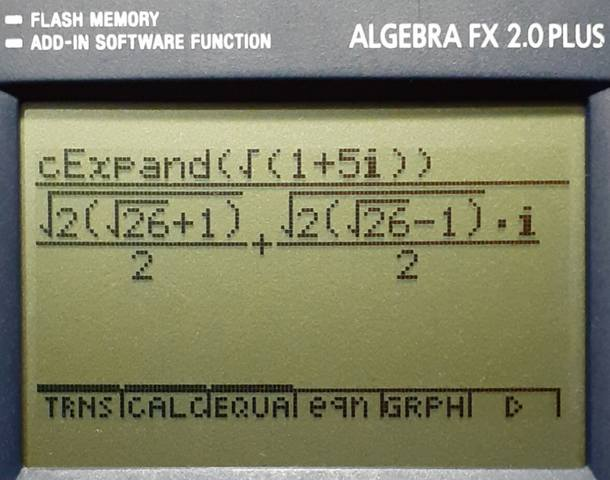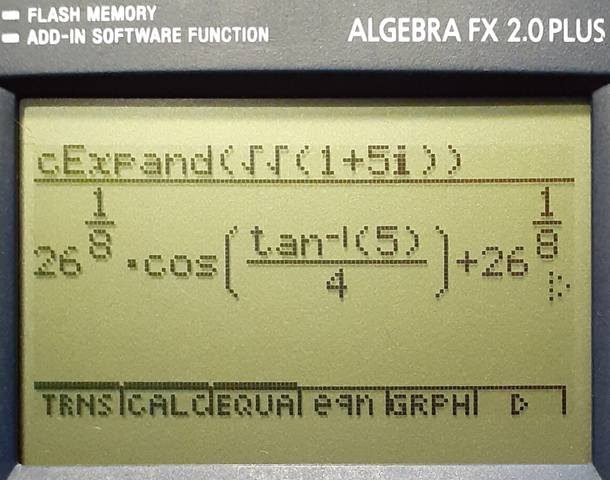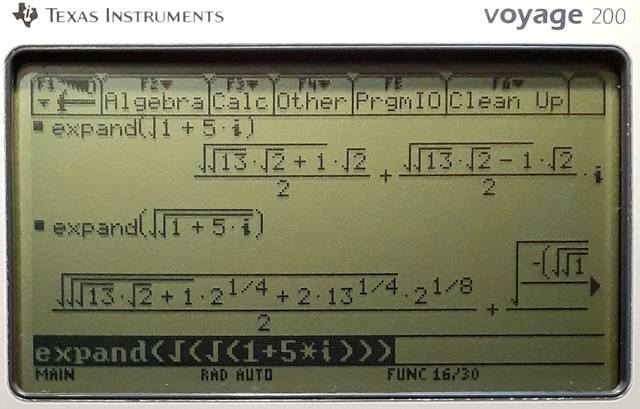sqrt(1+i)
09-26-2016, 12:35 PM (This post was last modified: 05-01-2018 11:16 PM by moonbeam.)
Post: #1
 moonbeamJunior Member Posts: 7 Joined: Feb 2016
sqrt(1+i)
(deleted by author)
09-26-2016, 06:19 PM
Post: #2
 parisseSenior Member Posts: 1,255 Joined: Dec 2013
RE: sqrt(1+i)
You must write your own program to do that. I have disabled exact sqrt of complex numbers on the Prime because it could freeze the calculator too easily (computations with algebraic extension becoming much too complicated and difficult to interrupt).
09-26-2016, 08:33 PM
Post: #3
 Helge GabertSenior Member Posts: 467 Joined: Dec 2013
RE: sqrt(1+i)
Can you re-write as

e^(1/2*LN(1+i)) ?

That would be consistent with (1+i)^(1/3), (1+i)^(1/4), etc. which result in

e^(1/3*LN(1+i)), e^(1/4*LN(1+i)), etc.

Better solution than to force an approximate result in CAS! Thanks.
09-26-2016, 08:38 PM
Post: #4
 dg1969Member Posts: 110 Joined: Apr 2014
RE: sqrt(1+i)
Math is not my favorite hobby all my excuse if the comment does not provide a real alternative...very strange... With
Code:
SQRT(1+i)
on the command line the prime provide an approx answer at the enter key press... but if you select the same "sqrt(1+i)" on the history (left side of the screen) by a touch screen and then press simplify...
09-27-2016, 04:19 AM
Post: #5
 Helge GabertSenior Member Posts: 467 Joined: Dec 2013
RE: sqrt(1+i)
Interesting. Thanks for the tip. The result is exact and correct.

But now try sqrt(sqrt(1+i)) . . . That is probably the reason why Bernard resorted to the approximations.
10-04-2016, 03:06 PM
Post: #6
 Helge GabertSenior Member Posts: 467 Joined: Dec 2013
RE: sqrt(1+i)
Interesting. If the TI-92 can do it, maybe that will provide an incentive for the HP Prime development team10-04-2016, 03:44 PM
Post: #7
 ErwinMember Posts: 166 Joined: May 2015
RE: sqrt(1+i)
(10-04-2016 12:41 PM)moonbeam Wrote:
(09-27-2016 04:19 AM)Helge Gabert Wrote:  But now try sqrt(sqrt(1+i)) . . . That is probably the reason why Bernard resorted to the approximations.

I concur that the exact results aren't likely to have practical use for all but the simplest cases and I recall from another thread that the Prime doesn't provide enough support to CAS to let it fail gracefully.

Having said that, I've installed the XCAS desktop app and sqrt(1+i) crashes it. I'm not sure if this is a bug or user error.

Getting intrigued, I tried it on my HP-50g. sqrt(1+i) works, sqrt(sqrt(1+i)) crashes it hard. My ancient TI-92Plus on the other hand just keeps chugging along when nesting sqrt functions; I got bored after eight...

Hi,

on a HP71B the result for sqr(sqr(1+j)): 1,06955393236 +0,212747504726j No problem with the ancient calculator :-) interesting, that ist could be a problem on the newer one - or I misunderstand the question
10-04-2016, 04:50 PM
Post: #8
 Helge GabertSenior Member Posts: 467 Joined: Dec 2013
RE: sqrt(1+i)
Yes, you are misunderstanding. The idea is to obtain an exact, symbolic result, please see above posts. The 71B does not have a built-in CAS.
10-04-2016, 06:34 PM
Post: #9
 parisseSenior Member Posts: 1,255 Joined: Dec 2013
RE: sqrt(1+i)
(10-04-2016 12:41 PM)moonbeam Wrote:  Having said that, I've installed the XCAS desktop app and sqrt(1+i) crashes it. I'm not sure if this is a bug or user error.
Strange, the current version of Xcas work with sqrt(1+i) and also sqrt(sqrt(1+i)) (but the answer is very complicated)
10-04-2016, 07:39 PM
Post: #10Tim WessmanSenior Member Posts: 2,290 Joined: Dec 2013
RE: sqrt(1+i)
(10-04-2016 03:44 PM)Erwin Wrote:  on a HP71B the result for sqr(sqr(1+j)): 1,06955393236 +0,212747504726j No problem with the ancient calculator :-) interesting, that ist could be a problem on the newer one - or I misunderstand the question

It is exclusively when dealing with exact, symbolic results (which keep getting larger and larger the more you apply them).

Prime returns the approximated result identically to the 71.

TW

Although I work for HP, the views and opinions I post here are my own.
10-05-2016, 05:34 AM
Post: #11Joe HornSenior Member Posts: 1,920 Joined: Dec 2013
RE: sqrt(1+i)
(10-04-2016 12:41 PM)moonbeam Wrote:  Getting intrigued, I tried it on my HP-50g. sqrt(1+i) works, sqrt(sqrt(1+i)) crashes it hard.

After 11.37 seconds, my 50g returns an exact result for 'sqrt(sqrt(1+i))' EVAL. Perhaps our settings differ?

<0|ɸ|0>
-Joe-
10-05-2016, 05:38 AM
Post: #12
 parisseSenior Member Posts: 1,255 Joined: Dec 2013
RE: sqrt(1+i)
(10-04-2016 07:35 PM)moonbeam Wrote:
(10-04-2016 06:34 PM)parisse Wrote:  Strange, the current version of Xcas work with sqrt(1+i) and also sqrt(sqrt(1+i)) (but the answer is very complicated)

The versions I downloaded are about ten days old. The examples above make it crash on the Windows box I used, but apparently not on a Mac. I haven't tried Linux.
I tried the windows 64, linux 64 and mac os versions without problems. Which version of xcas do you have?
Post: #13
 roadrunnerSenior Member Posts: 429 Joined: Jun 2015
RE: sqrt(1+i)
I created a little program to return the exact result for nested square roots of a complex number:

Code:
 #pragma mode( separator(.,;) integer(h32) ) rootroot(a,n); #cas rootroot(a,n):= BEGIN  local x,y,ra,ia;  ra:=RE(a);  ia:=IM(a);   y=√(-ra+√(ra^2+ia^2))/√2;  x=ia/(2*y);  if n==1 then return x+y*i; end;  return rootroot(x+y*i,n-1); END; #end

It crashes the emulator for values of n 6 or greater. Example:

rootroot(1+i,5) works but rootroot(1+i,6) crashes.

However, approx(rootroot(1+i,6)) returns the correct approximate answer, which tells me the program is returning a correct answer but the display can't handle it, and sits there with an hour glass in the corner.

What am I doing wrong?

-edited a grammatical error
10-05-2016, 01:52 PM
Post: #14
 parisseSenior Member Posts: 1,255 Joined: Dec 2013
RE: sqrt(1+i)
It has been reported that the windows 32 bits version of xcas works on windows 10 while the 64 bits version crashes.
10-05-2016, 05:54 PM (This post was last modified: 07-04-2017 02:46 PM by DedeBarre.)
Post: #15
 DedeBarreJunior Member Posts: 1 Joined: Oct 2016
RE: sqrt(1+i)
Hi..i am a new user here. In my case it crashes the emulator for values of n 6 or greater. rootroot(1+i,5) works but rootroot(1+i,6) crashes.However, approx(rootroot(1+i,6)) returns the correct approximate answer, which tells me the program is returning a correct answer but the display can't handle it, and sits there with an hour glass in the corner.

pcb assembly usa
10-05-2016, 07:24 PM (This post was last modified: 10-05-2016 07:34 PM by parisse.)
Post: #16
 parisseSenior Member Posts: 1,255 Joined: Dec 2013
RE: sqrt(1+i)
(10-05-2016 02:59 PM)compsystems Wrote:  I think the rules of simplifications on the ti68k calculators are more powerful in many cases
This is your opinion, I don't share it. As a counterexample try simplifying 4*atan(1/5)-atan(1/239) on your ti.
The handling of sqrt in Xcas is based on the more general concept of algebraic extension of Q (rootof in Xcas), where the emphasis is on recognizing 0 in a deterministic manner. Internally, there is no embedded sqrt inside Xcas, only a common algebraic extension for all the coefficients. Since displaying rootofs would scare many users, simple algebraic extensions are converted to more standard sqrt after computations, but as a side effect this does not necessarily *look* as simple (especially for embedded sqrt). And you can rework sqrt(1+i): for example if you want real or imaginary part: normal(re(sqrt(1+i))); normal(im(sqrt(1+i)))
10-06-2016, 01:03 PM (This post was last modified: 04-17-2017 12:53 AM by compsystems.)
Post: #17compsystemsSenior Member Posts: 1,342 Joined: Dec 2013
RE: sqrt(1+i)
(10-05-2016 07:24 PM)parisse Wrote:
(10-05-2016 02:59 PM)compsystems Wrote:  I think the rules of simplifications on the ti68k calculators are more powerful in many cases
.... As a counterexample try simplifying 4*atan(1/5)-atan(1/239) on your ti.
The handling of sqrt in Xcas is based on the more general concept of algebraic extension of Q (rootof in Xcas), where the emphasis is on recognizing 0 in a deterministic manner. Internally, there is no embedded sqrt inside Xcas, only a common algebraic extension for all the coefficients. Since displaying rootofs would scare many users, simple algebraic extensions are converted to more standard sqrt after computations, but as a side effect this does not necessarily *look* as simple (especially for embedded sqrt). And you can rework sqrt(1+i): for example if you want real or imaginary part: normal(re(sqrt(1+i))); normal(im(sqrt(1+i)))

ok, but they are more steps (input) to get to the same output,ti68k:
input (approx mode): √(1.0+i)
output x+y*i: 1.09868411347 + 0.455089860562*i

input (exact mode): √(1+i)
output x+y*i: sqrt(2*(sqrt(2)+1))/2 + (sqrt(2*(sqrt(2)+1))/2)*i

Xcas
input: normal(re(√(1+i))) + normal(im(√(1+i)))*i
output: (√2*√(√2+1)+(1+i)*√(√2+1))/(√2+2) not is a x + y * i form
fabulous if you include a flag in xcas to see the output of a complex expression in the form x + y * i Good Idea?

Xcas (next realease) =)
input (exact mode and new flag): √(1+i)
output: sqrt(2*(sqrt(2)+1))/2 + (sqrt(2*(sqrt(2)+1))/2)*i

another way to transform the output to x+y*i
√(1+i) -> (1+i)*√(√2+1)/(√2)
substituting i -> x
coeff( (1+x)*√(√2+1)/(√2),x) coeff separates the real and complex part
poly1[√2*√(√2+1)/2, √2*√(√2+1)/2]
Ans .* poly1[1, i]
poly1[√2*√(√2+1)/2, √2*√(√2+1)/2*i]
ΣLIST(poly1[√2*√(√2+1)/2, √2*√(√2+1)/2*i])
sqrt(2*(sqrt(2)+1))/2 + (sqrt(2*(sqrt(2)+1))/2)*i

we also need a version of QPI-ROOT cmd
Code:
QPIROOT(normal(re(√(1+i)))+normal(im(√(1+i)))*i)
-> sqrt(2*(sqrt(2)+1))/2 + (sqrt(2*(sqrt(2)+1))/2)*i
07-04-2021, 03:50 PM
Post: #18
 Albert ChanSenior Member Posts: 2,142 Joined: Jul 2018
RE: sqrt(1+i)
Code:
  y=√(-ra+√(ra^2+ia^2))/√2;  x=ia/(2*y);  if n==1 then return x+y*i; end;  return rootroot(x+y*i,n-1);  ...

It crashes the emulator for values of n 6 or greater. Example:

rootroot(1+i,5) works but rootroot(1+i,6) crashes.

However, approx(rootroot(1+i,6)) returns the correct approximate answer, which tells me the program is returning a correct answer but the display can't handle it, and sits there with an hour glass in the corner.

What am I doing wrong?

Code is wrong. Think polar form (cis(θ) = cos(θ) + i*sin(θ))

z = |z| * cis(arg(z))
√z = √|z| * cis(arg(z)/2)

With arg(z) = [-pi,pi], sign(arg(z)) = sign(sin(arg(z)) → sign(im(z)) = sign(im(√z))

CAS> csqrt(z) := √((abs(z)+re(z))/2) + sign(im(z))*i*√((abs(z)-re(z))/2)
CAS> csqrt(1+i)

$$\displaystyle \sqrt{\frac{1}{2} \cdot (\sqrt{2}+1)}+ i \sqrt{\frac{1}{2} \cdot (\sqrt{2}-1)}$$

CAS> csqrt(Ans)

$$\displaystyle i \sqrt{\frac{1}{2} \cdot (-\sqrt{\frac{1}{2} \cdot (\sqrt{2}+1)}+2^{\frac{1}{4}})}+\sqrt{\frac{1}{2} \cdot (\sqrt{\frac{1}{2} \cdot (\sqrt{2}+1)}+2^{\frac{1}{4}})}$$

CAS> approx(Ans) ﻿ ﻿ ﻿ ﻿ ﻿ ﻿ → 1.06955393236+0.212747504727*i
CAS> Ans^4 ﻿ ﻿ ﻿ ﻿ ﻿ ﻿ ﻿ ﻿ ﻿ ﻿ ﻿ ﻿ ﻿ ﻿ → 1+i

Also, with radical not simplified, mess exploded with nested square root.

CAS> rootroot(1+i, 1)

$$\displaystyle \frac{i \sqrt{\sqrt{2}-1}}{\sqrt{2}}+\frac{\sqrt{2} \cdot \frac{1}{2}}{\sqrt{\sqrt{2}-1}}$$

CAS> rootroot(Ans, 1)
$$\displaystyle \frac{1}{2} \cdot \frac{\sqrt{\sqrt{2}-1}}{\sqrt{\frac{1}{2} \cdot (2 \sqrt{\frac{1}{4} \cdot (\left(\frac{\sqrt{2}}{\sqrt{\sqrt{2}-1}}\right)^{2}+4 \left(\frac{\sqrt{\sqrt{2}-1}}{\sqrt{2}}\right)^{2})}-\frac{\sqrt{2}}{\sqrt{\sqrt{2}-1}})}}+\frac{i \sqrt{\frac{1}{2} \cdot (2 \sqrt{\frac{1}{4} \cdot (\left(\frac{\sqrt{2}}{\sqrt{\sqrt{2}-1}}\right)^{2}+4 \left(\frac{\sqrt{\sqrt{2}-1}}{\sqrt{2}}\right)^{2})}-\frac{\sqrt{2}}{\sqrt{\sqrt{2}-1}})}}{\sqrt{2}}$$

CAS> approx(Ans) ﻿ ﻿ ﻿ ﻿ ﻿ ﻿ → 1.06955393236+0.212747504727*i
07-05-2021, 05:31 PM
Post: #19HlibMember Posts: 242 Joined: Jan 2015
RE: sqrt(1+i)
I compared the calculated expressions √√...√(1+5×i) on the afx-2.0 and ti-v200 calculators.When the number of roots increases, afx-2.0 outputs a formula with trigonometric functions within 1.2_sec for any number of roots.Ti-v200 gives the result only with numbers and arithmetic operations. When the number of roots is eight ( √√√√√√√√ (1+5×i) ), v200 calculates the example for about 3 minutes 40 seconds (too long).In HP-Prime, it would be worth implementing automatic switching of the calculation method depending on the complexity of the final result and on the availability of free space in RAM (not only in this example).
Post: #20
 roadrunnerSenior Member Posts: 429 Joined: Jun 2015
RE: sqrt(1+i)
(07-04-2021 03:50 PM)Albert Chan Wrote:  Code is wrong. Think polar form (cis(θ) = cos(θ) + i*sin(θ))

That works a lot better. Revision B of rootroot:

Code:
#pragma mode( separator(.,;) integer(h32) ) rootroot(a,n); #cas rootroot(a,n):= BEGIN  n:=trunc(n);  if n<=0 then return undef; end;  csqrt:=√((abs(a)+re(a))/2)+sign(im(a))*i*√((abs(a)-re(a))/2);  if n==1 then return csqrt; end;  return rootroot(csqrt,n-1); END; #end

Thank you sir!